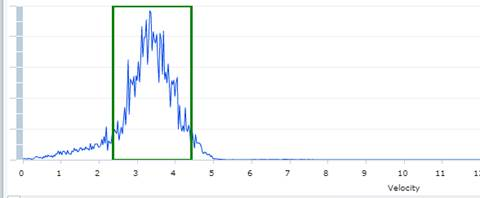# What does the generated graph mean when you take a diagnostic reading (sample) on a FLO-DAR mean?

## Document ID

Document ID TE8958

## Published Date

Published Date 11/08/2017
Question
What does the generated graph mean when you take a diagnostic reading (sample) on a FLO-DAR mean?
Summary
How to understand the graph generated when taking a diagnostic reading (sample) on a FLO-DAR.
1. Below is an example of the curve generated by a FLO-DAR.
• This curve is only related to the velocity measurement.1. How the FLO-DAR takes a velocity reading.
• The FLO-DAR takes a large number of velocity readings for each measurement.
• The returns from the readings are then sent through an FFT algorithm (Fast Fourier Transform).   The result is the curve that you see when you take a sample.
2. Description of the curve
• The horizontal axis is the velocity
• The vertical axis is the number of occurrences
• The peak is where there are the most velocity readings.
• A good FFT curve a one peak that is well defined and not too wide.
• The above peak is a good peak, although it is slightly wide.
3. Note the "green box" around the peak of the curve.  The FLO-DAR has an algorithm that looks at this curve and picks the area where the peak is.  It marks this area with the green box.
• On the FLO-STATION and FL1500, instead of putting a green box around the area, it changes the curve to yellow.
4. The FLO-DAR then analyzes the velocity points inside the green box to give the Surface Velocity reading.  In the above example, the Surface Velocity was calculated to be 3.30 fps (feet per second).
5. The FLO-DAR then uses another algorithm to calculate the average velocity for the site.
• The Surface Velocity is an independent measurement.
• The Average Velocity is dependent on the geometry of the site:
• The pipe shape and dimensions
• The Level of the water in the pipe.
• For example, in a round pipe the Average Velocity calculation depends on:
• The Pipe Diameter
• The Level measurement